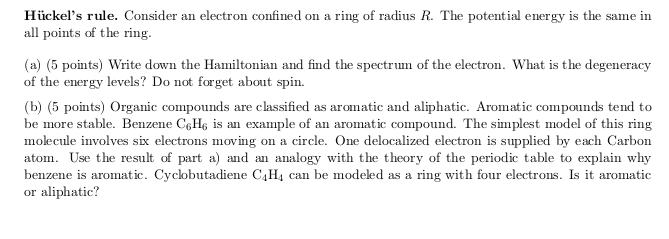# Quantum degeneracy problem, electron on a ring

VortexLattice

## Homework StatementBelow

## The Attempt at a Solution

So this is a lot like the infinite square well, except periodic. If S is an arc length, then $S = \theta R$ so $\frac{d^2}{dS^2} = \frac{1}{R^2}\frac{d^2}{d\theta^2}$, which is more convenient to use in the hamiltonian. So for the hamiltonian I get:

$$H = \frac{-\hbar^2}{2m}\frac{1}{R^2}\frac{d^2}{d\theta^2} + V_0$$

With Schrodinger's equation, I get

$$\frac{d^2 \psi^2}{d\theta^2} = -\frac{2mR^2(E - V_0)}{\hbar^2}\psi$$

Which gives solutions of the form $\psi = \frac{1}{\sqrt{2\pi}}e^{\pm i k \theta}$, where $k = \sqrt{\frac{2mR^2(E - V_0)}{\hbar^2}}$.

Then, because it's a ring, we need $\psi(x + 2\pi) = \psi(x)$ for any x, which gives us the requirement that k is an integer. So our energy levels are $E = \hbar^2 k^2/2mR^2 + V_0$, and it seems like they have a degeneracy of 4 because we have two functions for each k with the same energy, and then for each of them, the electron's spin can be up or down. Is that right?

As for part (b), I have no idea... Benzene has 6 free electrons, so according to my degeneracy, it completely fills the first energy level, and then there are 2 electrons in the 2nd energy level. Ok...then they ask about a compound with 4 electrons. This seems like it just fills the first energy level, but that seems too simple and stupid to be right.

Can anyone help me out?

Thanks!

Homework Helper
Gold Member
k is an integer including zero. What is the degeneracy of the energy level corresponding to k = 0?

VortexLattice
k is an integer including zero. What is the degeneracy of the energy level corresponding to k = 0?

Well I guess that just has a degeneracy of 2 due to the spin, right?

Any idea on the aromatic thing? I'm totally clueless about that...

VortexLattice
Ah, I think I see, after what you just said and reading the wiki article on Huckel's Rule... The number of states for k =/= 0 is 4 for each energy level. For k = 0 it's 2. So like Huckel's Rule, for energy level n, there are 4n + 2 states. So benzene is aromatic because it has 6 electrons, so fully completes the n = 1 energy level. The other compound has 4 however, so it fills the n = 0 level but only half fills the n = 1 level, so it's not stable (though from the little I know of chemistry, I thought that just meant it's more reactive, not less stable).

Homework Helper
Gold Member
I think your analysis is now correct. I'm not very clear on the reactive vs. stable interpretation either. Maybe someone can clarify it.